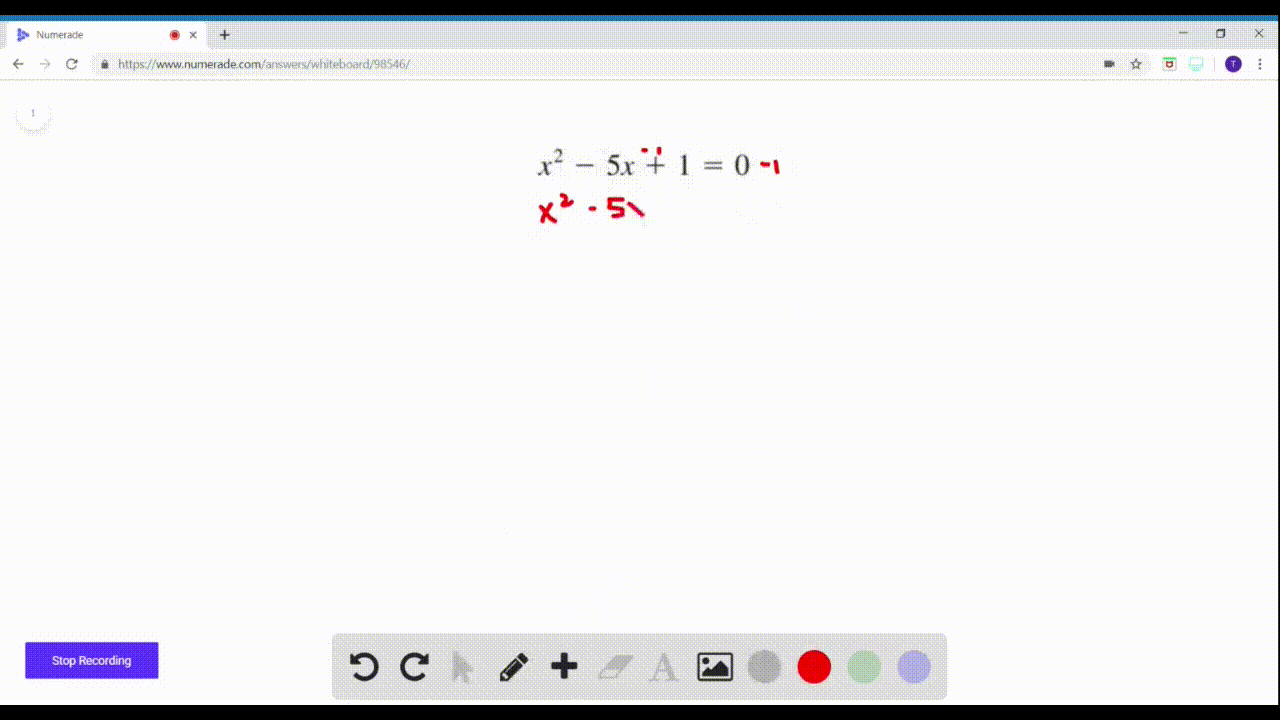Enroll in one of our FREE online STEM summer camps. Space is limited so join now!View Summer Courses### $17-28=$ Completing the Square Find all real solu…

02:27Central Michigan University

Need more help? Fill out this quick form to get professional live tutoring.

Get live tutoring
Problem 21

$17-28=$ Completing the Square Find all real solutions of the
equation by completing the square.
$$x^{2}+x-\frac{3}{4}=0$$

$x=\frac{1}{2}$ and $x=-\frac{3}{2}$

## Discussion

You must be signed in to discuss.

## Video Transcript

Okay, completing the square. We've got X squared plus X plus three. Force equal zero. We're gonna complete this Our solve this by completing the square. So the first thing we do is we subtract, um, three force from both sides to get the equation in this format. Then we do what's called completing the square. We try to turn this left. The stars are the expression on the left side of this, um, into a perfect square. Try no meal. We do that by taking half of this value right here. This be value the coefficient of the ex term, which is currently one. So half of that would be 1/2 and then we square it. 1/2 squared is 1/4 so we add 1/4 to both sides. The reason we do that is because then this try no, me over here on the left is a perfect square. Try no meal that can be factored like this. So, um, X squared plus x plus. 1/4 is the same as X plus, 1/2 squared, and then on the right, we end up with, um you know what it is the original expression. Wrong. So I'll fix this so I could change two months, though. It was supposed to be subtraction. So then when we we were actually adding three forced to both sides. So there we go. So now it's everything's correct. Now we can do three force plus one force on the right, which is one. Okay. Now, the reason we factored was so that we could take the square root of both sides and start solving for X. So the square root of X plus 1/2 squares just experts 1/2 the square root of one is plus or minus one. And then, um, subset of some more room. Here we subtract 1/2 from both sides and we'll start seeing a picture of our answer. Here s O X equals negative 1/2 plus or minus one. I'm gonna think of one as two halfs right here so that I have a common denominator and combined these together so negative 1/2 minus two halves is negative. Three halfs. That's one of our answers. And negative 1/2 plus two halves is 1/2. That's our other answer. So these are the two solutions to the original equations that we found by completing the square